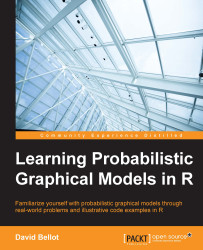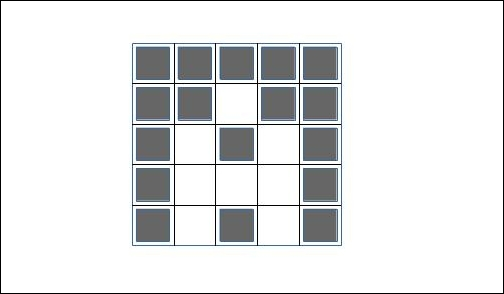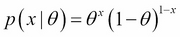•#### Learning Probabilistic Graphical Models in R#### Overview of this book

Probabilistic graphical models (PGM, also known as graphical models) are a marriage between probability theory and graph theory. Generally, PGMs use a graph-based representation. Two branches of graphical representations of distributions are commonly used, namely Bayesian networks and Markov networks. R has many packages to implement graphical models. We’ll start by showing you how to transform a classical statistical model into a modern PGM and then look at how to do exact inference in graphical models. Proceeding, we’ll introduce you to many modern R packages that will help you to perform inference on the models. We will then run a Bayesian linear regression and you’ll see the advantage of going probabilistic when you want to do prediction. Next, you’ll master using R packages and implementing its techniques. Finally, you’ll be presented with machine learning applications that have a direct impact in many fields. Here, we’ll cover clustering and the discovery of hidden information in big data, as well as two important methods, PCA and ICA, to reduce the size of big problems.
Learning Probabilistic Graphical Models in RCreditswww.PacktPub.comPrefaceFree Chapter
Probabilistic ReasoningExact InferenceLearning ParametersBayesian Modeling – Basic ModelsApproximate InferenceBayesian Modeling – Linear ModelsProbabilistic Mixture ModelsAppendixIndex## Mixture of Bernoulli

The mixture of Bernoulli is another interesting problem. As we saw earlier, the graphical model to represent such a model is always the same. Only the probability distribution functions associated to each node change compared to the previous example. The mixture of Bernoulli finds applications in particular in analyzing black and white images, where an image is made of one Bernoulli variable for each pixel. Then the goal is to classify an image, that is, to say which value of the latent variable produced it, given some observed pixels.

For example, the following (very stylized) figure represents the letter A:In a real application, we would use more pixels. But the idea of the application is to associate the value of pixels to each value of the latent variable, each one representing a different letter. This is a simple model for character recognition.

The distribution of a Bernoulli variable is:Now, let's say we have D Bernoulli variables. Each Bernoulli variable is parameterized...